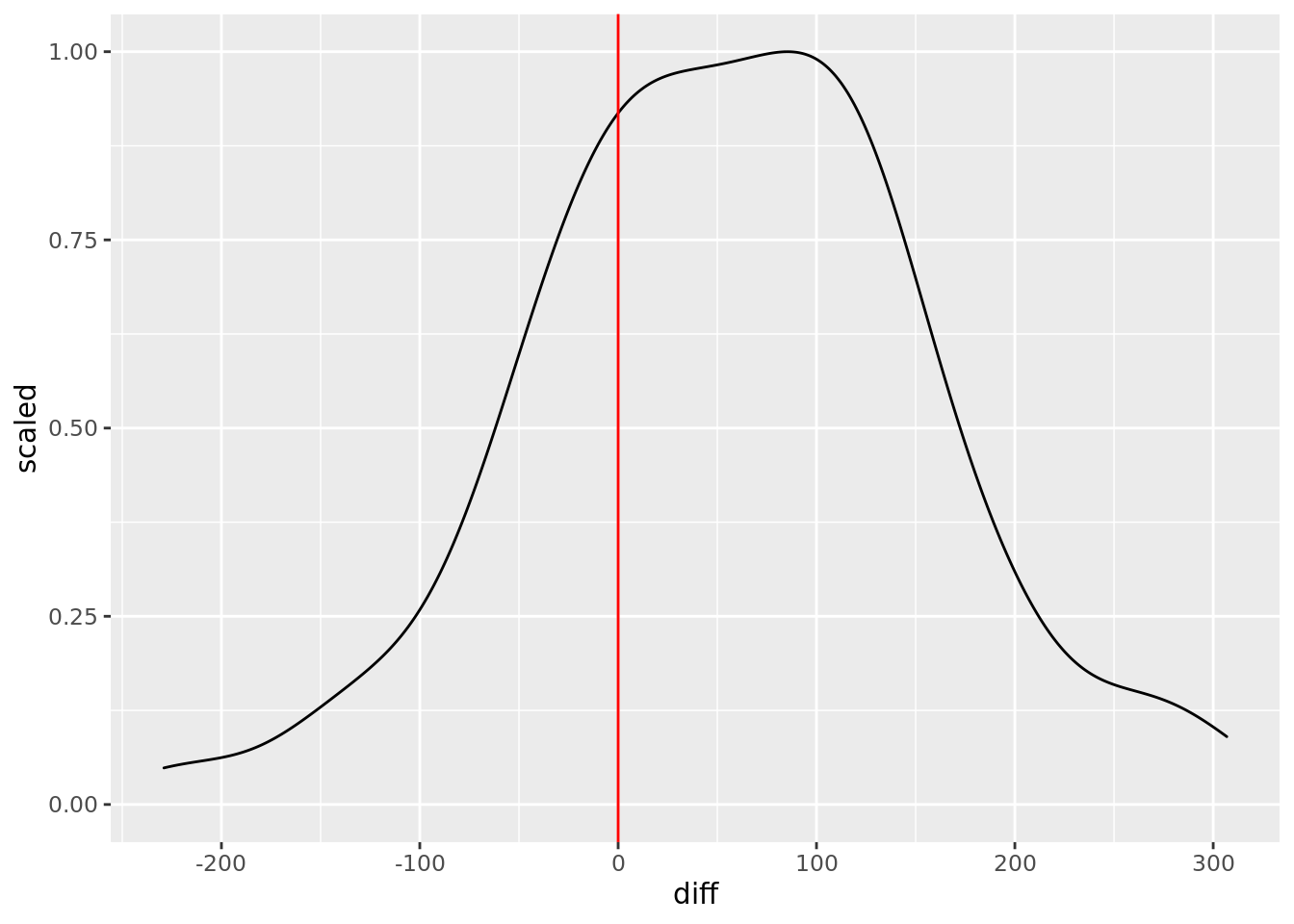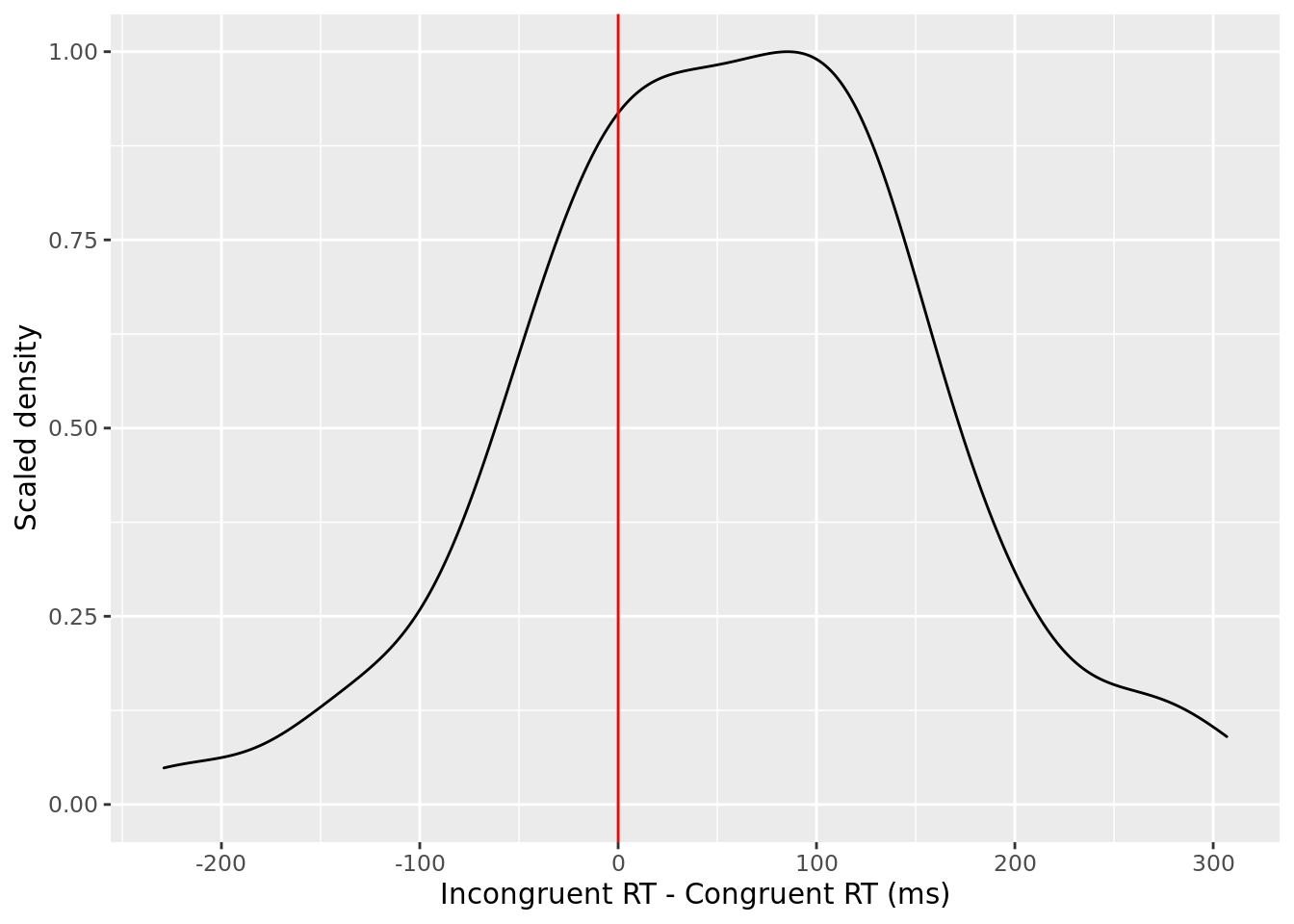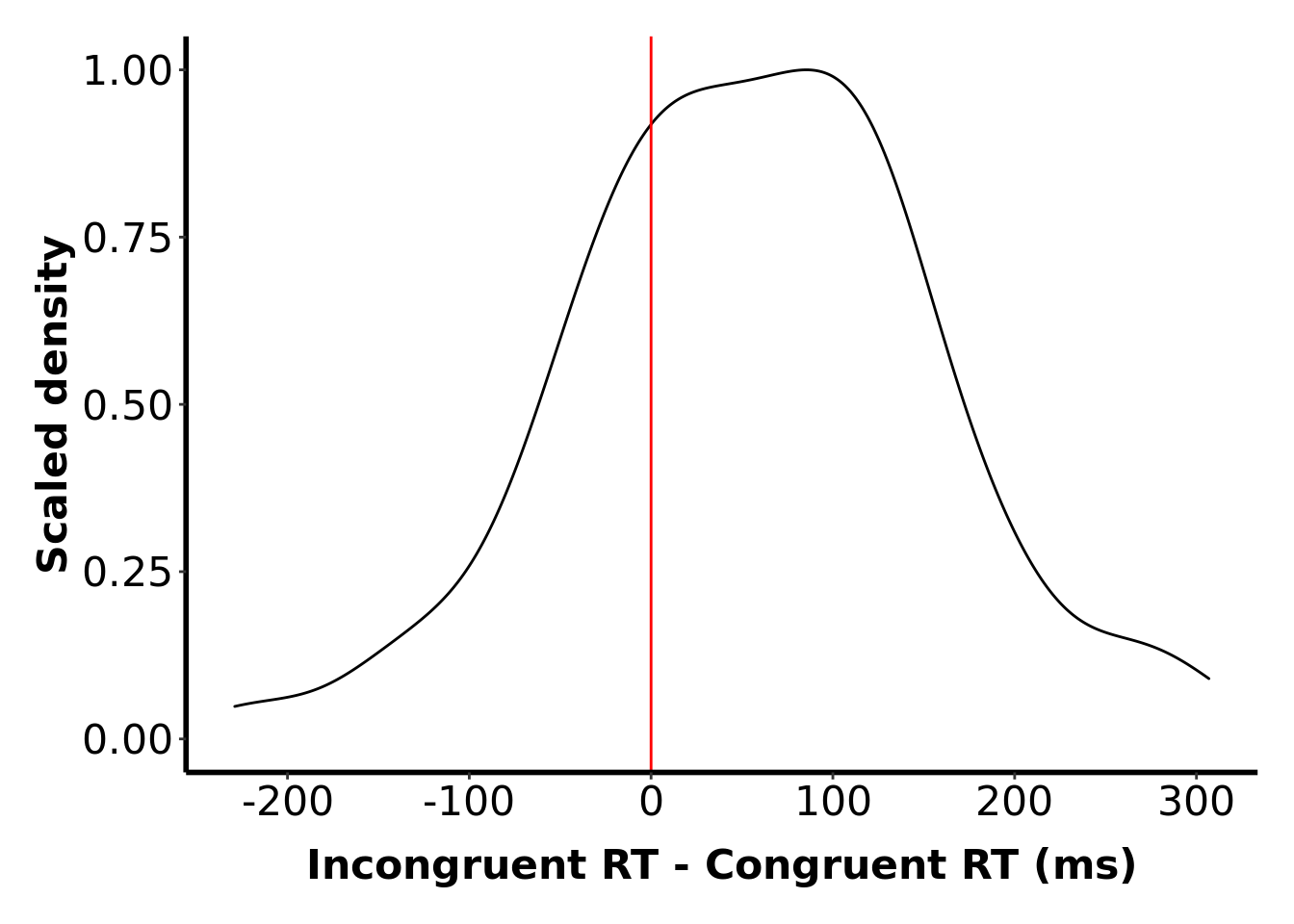# Before you start

1. Open the `rminr-data` project we used previously.

2. Ensure you have the files required for this worksheet, by asking git to “`pull`” the repository. Select the `Git` tab, which is located in the row of tabs which includes the `Environment` tab. Click the `Pull` button with a downward pointing arrow. A window will open showing the files which have been pulled from the repository. Close the `Git pull` window.

# Introduction

In this worksheet, we’ll look at how to produce publication-quality graphs in R. We start with an example from a previous worksheet.

In the exercise for the within-subject differences worksheet, we produced a density plot of the within-subject differences in reaction time for congruent versus incongruent trials. Let’s pick up where we left off. Go back to your `rminr-data` project, and open up the R file you used for this exercise. If you didn’t complete that exercise, or can’t find the file, you can get a copy here.

After some preprocessing, the graphing command we used in that exercise was as follows.

Enter this command into your script, and run it:

``````ctrldiff %>% ggplot(aes(diff)) +
geom_density(aes(y=..scaled..)) +
geom_vline(xintercept = 0, colour = 'red')``````This graph looks OK, but would need some improvement before including in a report or journal article. Here are the steps of this makeover.

# Meaningful labels

The first thing to do is change the axis labels to something a bit more human readable. We use the `xlab` and `ylab` commands for this. We covered these commands previously in the Absolute Beginners’ guide.

Update the commands in your script to look like this, and re-run them:

``````ctrldiff %>% ggplot(aes(diff)) +
geom_density(aes(y=..scaled..)) +
geom_vline(xintercept = 0, colour = 'red') +
xlab("Incongruent RT - Congruent RT (ms)") +
ylab("Scaled density")``````# Journal styling

The default styling for `ggplot` is different to what is preferred in most psychology journals. Fortunately, we can use Tina Seabrooke’s `theme_APA` to correct this. You’ll find the code in the same git repository as the data, so all you need to do is load in her code.

Enter this command at the top of your your script, and run it:

``source("themeapa.R")``

and then add it as a theme to your graph (much as you have used `theme_bw` in the past).

Update the commands in your script to look like this, and re-run them:

``````ctrldiff %>% ggplot(aes(diff)) +
geom_density(aes(y=..scaled..)) +
geom_vline(xintercept = 0, colour = 'red') +
xlab("Incongruent RT - Congruent RT (ms)") +
ylab("Scaled density") +
theme_APA``````# High-quality output

If you are writing a report or journal article, it’s generally a bad idea to screenshot your graphs, and it’s also generally a bad idea to use the export functionality within Rstudio. This is because both of these options produce graphics that are not high enough quality for publication.

To produce high-quality output, you should first create an object for your graph, much as we created an object for the output of analysis.

Update the commands in your script to look like this, and re-run them:

``````dgraph <- ctrldiff %>% ggplot(aes(diff)) +
geom_density(aes(y=..scaled..)) +
geom_vline(xintercept = 0, colour = 'red') +
xlab("Incongruent RT - Congruent RT (ms)") +
ylab("Scaled density") +
theme_APA
dgraph``````We can now use the `ggsave` command to save a high-quality version of that graph.

Enter this command at the bottom of your script, and run it:

``````ggsave(filename = "fig1.pdf", plot = dgraph, units = "cm", width = 15,
height = 10)``````

## Explanation of command

`filename = "fig1.pdf"` - Save the graph as fig1.pdf. Try to use PDF where possible, because it produces the best quality output and the smallest file size. However, if you’re unfortunate enough to be using a wordprocessor than cannot import PDF graphs (e.g. Microsoft Word) then you can use PNG format instead. You do this by changing the filename, e.g. `filename = "fig1.png"`. If you send your paper to a journal for consideration, they will also require the PDF version of your graphs as a separate attachement, as PNG files are generally not good enough for professional publications. For most internal reports (and university coursework), PNG is generally good enough.

`plot = dgraph` - Use the object called `dgraph` as the graph you want to save.

`units = "cm"` - The following commands will set the size of the graph; this command says what units these are in. You usually want to use “cm” (centimetres) but if you live in a country that hasn’t adopted the metric system, you can use “in” (inches) instead.

`width = 15` - The graph (including border etc.) should be 15 units wide (the units in this case being centimetres)

`height = 10` - The graph (including border etc.) should be 10 units high (centimetres in this case).

## Explanation of output

A file called fig1.pdf will have appeared in your Files window in RStudio. You can export this in the usual way.

# Choosing a graph for your data

So, now you know how to create a professional-quality graph in R, but which type of graph best suits your data? A graph should visually describe patterns in data that would otherwise be difficult to communicate. Choosing and configuring the most appropriate graph for you data will depend on what you want to communicate to your reader. In practice, making this decision often involves trying out different types of graph, and the elements used to build them. You might generate new ideas for graphs after you have analysed your data and begun to interpret the results.

These subjective aspects make it difficult to provide hard and fast rules for the type of plot you should choose, and the elements that it should contain. A general piece of advice is to carefully consider the best way to represent the centre (often the mean), and distribution of your data. We present some suggestions for plotting different types of data in the following sections.

As a side note, a common criticism of student projects is that results sections don’t include a graph, and appendices often contain lots of graphs that don’t really contribute to the report. You can overcome this by learning to experiment with different ways of plotting your data. When you’ve found a graph that contributes to the argument you’re trying to make, be confident and include it in your results section! Appendices are seldom read.

The rest of this worksheet gives a variety of examples of graphs one can produce in R. The examples have been categorized on the following criteria:

• Design type: Does your hypothesis involve a within subjects manipulation, a between-subjects manipulation, or a correlation between variables?

• Design complexity: Does your hypothesis involve a single factor, or an interaction between two factors?

• Variable type: If independent variables / predictor variables have more than two levels, are those levels ordered or unordered? For example, age is an ordered variable, but gender is an unordered variable.

This may help you narrow down the range of possibilities to those most suitable to the question you wish to investigate.

Another way we can categorize plots is by the sort of graphical device we use (e.g. lines, bars, distributions). So, if you’re looking to do a particular sort of plot, these examples can also help – not only on how to produce that plot, but also to think about when such a plot is a good choice.

The examples in this worksheet are just a small sample of what can be achieved in R; for much more, take a look at the excellent R Graph Gallery.

# Within-participants manipulations

We’ll start by producing some graphs for within-participants manipulations.

## Single factor, ordered variable

Our first example uses data from an undergraduate student experiment on the Perruchet Effect. Stay in your `rminr-data` project, create a new R file called `one-within.R`, and enter the commands that follow into that file.

To get started on this example, we need to first load the data.

Enter these commands into your script, and run them:

``````library(tidyverse)
The data we’ll focus on here are the mean expectancy ratings (`expect`) made by each participant (`subj`) for each of three Levels of the within-subjects factor (`level`). It’s not critical to understand what the within-subjects factor is here, and it would take a while to explain, but take a look at this worksheet for full details if you are curious. The main point to appreciate is the this is a within-subjects manipulation, so each participant provided data at each Level.
Our aim is to plot a graph for this single, within-participants factor called `level`. The simplest graph we could produce here would be to just plot the three means, but, when we graph data from psychology experiments, we generally try to give an indication of the variability between participants - is everyone exactly like the mean, or do people differ? One common way of doing this it to plot some kind of ‘error bar’; for example, as shown in Figure 1 of McAndrew et al. (2012). McAndrew et al. are not clear how they calculated these bars, but it’s quite likely that they are the standard errors, considering each Level separately – because this is the most common plot of this type. Such error bars are not particularly informative because, for example, they represent the variability between participants at each Level, when the experiment is a repeated measures design and so it is the variability of the trends across Level that is most relevant.